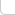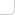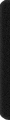# 某岛

… : "…アッカリ～ン . .. . " .. .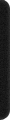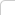October 6, 2021

# 传送门

(据说这场 8 个题才算签到。。可怕。。。)

# Problem D. Gene Folding

（就像是我们在最优子矩形里，用单调栈处理出以 i 位置为最小值，向左和向右最远可以延伸到哪里一样）

# Problem F. Ley Lines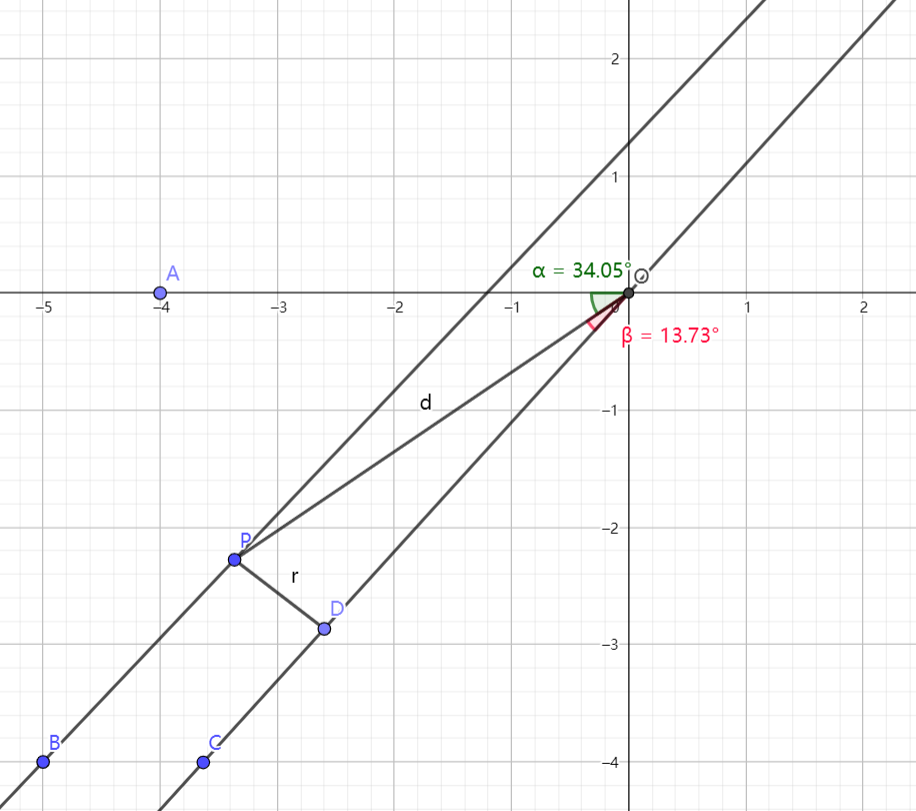const int N = int(3e3) + 9;
Po P[N];
int n, r;

int main(){

#ifndef ONLINE_JUDGE
//freopen("in.txt", "r", stdin);
//freopen("/Users/minakokojima/Documents/GitHub/ACM-Training/Workspace/out.txt", "w", stdout);
#endif

RD(n, r); REP(i, n) P[i].in();

int z = 2; REP(a, n) {
vector<pair<DB, int>> E;
int s = 1; REP(b, n) if (b != a){
Po v = P[b] - P[a]; DB d = v.len();
DB a = v.arg();
E.PB({a, 1}); E.PB({a + PI, -1});
if (d > r) {
DB b = asin(r/d);
E.PB({a + b, -1});
E.PB({a + PI - b, 1});
}
}
SRT(E); for (auto e: E) checkMax(z, s += e.se);
}
cout << z << endl;
}


# Problem J. ‘S No Problem

dp[u] 表示当前节点的奇偶性，以及子树中一共出现了多少路径的端点，简单转移即可。

# Problem B. The Cost of Speed Limits

————————

dp[u][i] 表示将这个节点相邻的边全部修改成 i 的代价，
sign[u] 表示所有相邻节点防止限速牌的代价，
best[u] 表示所有 dp[u] 和 sign[u] 的最小值。

sign[u] = c*SZ(adj[u]) + \sum best[v]
dp[u][i] = \sum min(sign[v] + 修改到 v 的边权, dp[v][i]) + 修改到 p 的边权

# Problem H. QC QC

atcoder 也出过一个版本

1. 真预言家报查杀。
2. 狼人悍跳诬陷预言家。
3. 狼人出卖自己的狼同伴以做高自己的身份。

# Problem N. What’s Our Vector, Victor?